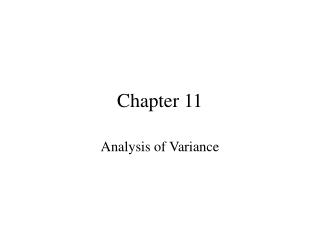Download PresentationChapter 11# Chapter 11 - PowerPoint PPT Presentation

Download Presentation##### Chapter 11

Download Policy: Content on the Website is provided to you AS IS for your information and personal use and may not be sold / licensed / shared on other websites without getting consent from its author. While downloading, if for some reason you are not able to download a presentation, the publisher may have deleted the file from their server.

- - - - - - - - - - - - - - - - - - - - - - - - - - - E N D - - - - - - - - - - - - - - - - - - - - - - - - - - -
##### Presentation Transcript

1. Chapter 11 Analysis of Variance

2. 11.1: The Completely Randomized Design: One-Way Analysis of Variance • vocabulary • completely randomized • groups • factors • levels • experimental units, • response

3. Analysis of Variance • Total Variation: the sum of squared differences between each measurement and the overall average (or grand mean). • You can divide Total Variation into parts. Today we use 2 parts: Among-Group and Within-Group. • SST= SSA + SSW

4. ANOVA • If a sum of squares is divided by the appropriate degree of freedom, it becomes a variance. Thus, ANOVA. • We use these variances in the “F” test for Differences in More than 2 Means. • The results are usually presented in an ANOVA summary table. • The “F” test has the same parts as previous hypothesis tests.

5. Example: Problem 11.8 • What is the factor? • What are the levels? • What are the hypotheses? • What is the decision rule? • What is the test statistic? • What is the “p” value? • What can we conclude?

6. Post hoc Testing or Multiple Comparisons • If at least 1 of the means is different, the next question is: which one(s) are significantly different? • Use the Tukey-Kramer Procedure: • calculate the “critical range” (11.6) • calculate the absolute differences for all pairs of means • Any absolute difference bigger than the critical range signals a significant difference.

7. Post hoc testing on 11.8 • Critical range shows that experts differs from readers and that readers differs from darts. • Note that more groups implies a lot more pairs. • text example: 4 goups: 6 pairs (page 404)

8. ANOVA Assumptions • Randomness and Independence • Normality • How do we test this? • Homogeneity of Variance • Levine Test: uses medians. • Understand existence but not mechanics.

9. 11.2: The Randomized Block Design • Do 11.1 again(!) except do it with blocks. • What is a block? • Sets of experimental units that have been matched. • Experimental units that have repeated measurements performed on them. • VERY SIMILAR to dependent means “t” test.

10. Theory • Take SST and repartition it. • SSA, SSBL, and SSE. • By doing this, you have increased calculated “F” because “F” is now SSA/SSE, and SSE is less than SSW. • Thus, the experiment is more sensitive—it does a better job of discovering or revealing factor effects.

11. Practice • Little has changed from the 1-way ANOVA: • The hypotheses are the same. • You are interested in the main factor, not the blocking factor. • The ANOVA table has an additional row for the Blocking source of variation. • Use Excel or Minitab.

12. Notes • You can test the block effects but most statisticians do not report the results. The test will tell how useful blocking is. So will RE. • The improvement in sensitivity is known as an improvement in Relative Efficiency (RE). • We will not cover Relative Efficiency further. • Post hoc testing is available and we will cover it.

13. Post hoc Testing • Use the Tukey procedure. • Equation 11.15.

14. 11.3: The Factorial Design: Two-Way Analysis of Variance • Similar to section 11-1 except uses 2 factors to partition variation. • Think of the 2 factors as your 2 ways to account for the inherent variability in the data. • Use software to do the math. • The new wrinkle is interaction.

15. Theory • This time, you have 2 independent factors. • Take SST and (yet again) repartition it. • SST = SSA + SSB + SSAB + SSE • SSA and SSB result from imposing your 2 factors (Factor A and Factor B) on the variability that lives in the data set.

16. In Practice with 2 Factors Check for Interaction First! • What is interaction? Interaction occurs when the level of Factor A affects or determines how the data react to Factor B (and vice versa). • Look at the plot. • H0: interaction equals 0 (no I/A). • H1: interaction is not equal 0 (I/A exists).

17. When you find significant interaction • If reject H0, don’t bother checking for significant A and B Factor effects. • Conduct Post hoc testing for significantly different combinations of A and B. • Post hoc testing for interaction involves more Tukey testing which is not presented in this text.

18. When there is no significant interaction: • Examine the Main Effects. • This examination is most often done by using the appropriate “F” test. • It can also be done graphically--informally. • If there is no interaction and there are 3 or more levels of the significant factor, post hoc testing is possible.